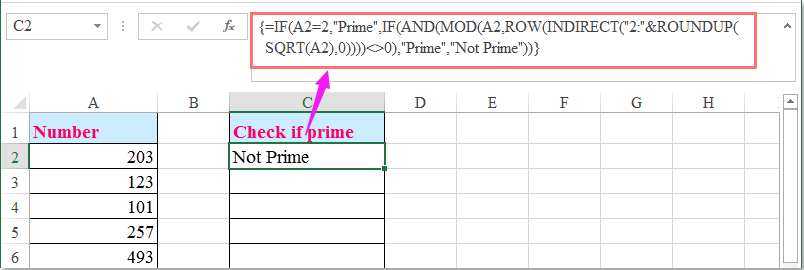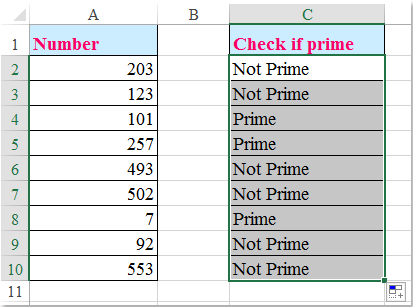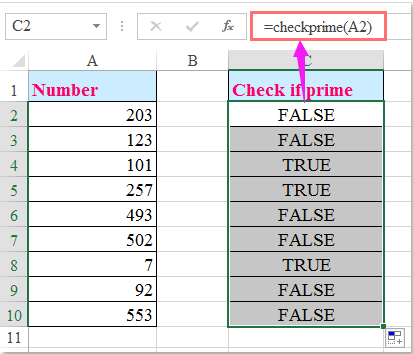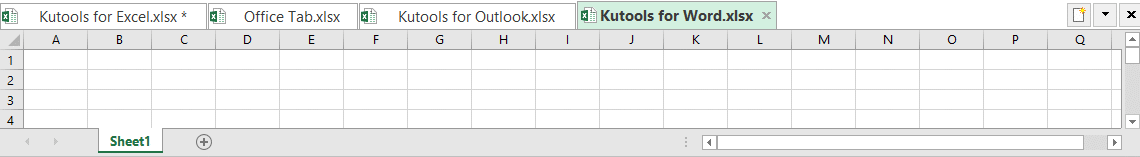oror

## 如何检查数字是Excel中的素数？

####检查数字是否是数组公式的素数###### 节省50％的时间，每天为您减少数千次鼠标点击！

1。 在空白单元格中输入以下公式 - 例如在数据旁边的C2：

=IF(A2=2,"Prime",IF(AND(MOD(A2,ROW(INDIRECT("2:"&ROUNDUP(SQRT(A2),0))))<>0),"Prime","Not Prime")) (A2 是单元格包含您想要检查的号码），然后按 Ctrl + Shift + Enter 如果数字为素数，则会在单元格中显示“Prime”，否则将显示“Not Prime”，请参阅屏幕截图：2。 然后选择单元格C2，然后将填充手柄向下拖动到您要应用此公式的单元格，如果是素数，则所有数字都将被标识。 看截图：####用用户定义函数检查一个数字是否是质数

1。 按住 ALT + F11 键打开 Microsoft Visual Basic for Applications 窗口。

2。 点击 插页 > 模块，并将以下代码粘贴到 模块窗口.

VBA代码：检查数字是否为素数：

```Function CheckPrime(Numb As Single) As Boolean
'Updateby Extendoffice 20160614
Dim X As Long
If Numb < 2 Or (Numb <> 2 And Numb Mod 2 = 0) _
Or Numb <> Int(Numb) Then Exit Function
For X = 3 To Sqr(Numb) Step 2
If Numb Mod X = 0 Then Exit Function
Next
CheckPrime = True
End Function
```

3。 然后保存并关闭此代码，返回到工作表，并输入此公式： = checkprime（A2） 进入数字列表旁边的空白单元格，然后将填充手柄向下拖到要包含此公式的单元格中，如果数字是质数，则它将显示TRUE，否则将显示FALSE，请参见截图：#### Kutools for Excel - 最佳办公生产力工具提高80％的生产力

• 重用: 快速插入 复杂的公式，图表 以及你以前用过的任何东西; 加密单元格 密码; 创建邮件列表 并发送电子邮件...
• 超级方程式酒吧 （轻松编辑多行文字和公式）; 阅读布局 （轻松读取和编辑大量单元格）; 粘贴到过滤范围...
• 合并单元格/行/列 不丢失数据; 分裂细胞含量; 组合重复的行/列...防止重复的细胞; 比较范围...
• 选择复制或唯一 行; 选择空行 （所有细胞都是空的）; 超级查找和模糊查找 在许多工作簿中; 随机选择......
• 精确复制 多个单元格而不更改公式参考; 自动创建参考 多张表; 插入项目符号，复选框等等......
• 提取文本，添加文本，按位置删除， 删除空间; 创建和打印分页小计; 在单元格内容和注释之间转换...
• 超级过滤器 （将过滤方案保存并应用到其他工作表）; 高级排序 按月/周/日，频率等; 特殊过滤器 用粗体，斜体......
• 结合工作簿和工作表; 根据键列合并表; 将数据拆分为多个表格; 批量转换xls，xlsx和PDF...
• 超过300强大的功能。 支持Office / Excel 2007-2019和365。 支持所有语言。 在您的企业或组织中轻松部署。 全功能60天免费试用。#### Office选项卡为Office提供选项卡式界面，使您的工作更轻松

• 在Word，Excel，PowerPoint中启用选项卡式编辑和阅读，Publisher，Access，Visio和Project。
• 在同一窗口的新选项卡中打开并创建多个文档，而不是在新窗口中。
• 通过50％提高您的工作效率，每天为您减少数百次鼠标点击！Say something here...
symbols left.
###### or post as a guest, but your post won't be published automatically.
Loading comment... The comment will be refreshed after 00:00.
• To post as a guest, your comment is unpublished.
· 2 months ago
Yes it worked wen I did Ctrl+Shift+Enter... !! Thank you..:D and Do u mind explaining the algorithm please.
• To post as a guest, your comment is unpublished.
· 4 months ago
There's a reference error when you type in numbers that have more than 12 digits.
• To post as a guest, your comment is unpublished.
· 3 months ago
Hello,Nathan,
As you said, when the numbers are longer than 12 digits, it will become scientific notation. The formula is not applied for this formatting.
• To post as a guest, your comment is unpublished.
· 8 months ago
well 4095 is not a prime. still it gives result as prime no though. its incorrect.
• To post as a guest, your comment is unpublished.
· 8 months ago
Hello, vaibhav,
When you pasting above formula, you should press Ctrl + Shift + Enter keys together, not just Enter key, please try it again.
• To post as a guest, your comment is unpublished.
· 1 years ago
Your formula is not working. Eg. 1681 is NOT a prime but by formula it is.
=IF(A2=2,"Prime",IF(AND(MOD(A2,ROW(INDIRECT("2:"&ROUNDUP(SQRT(A2),0))))<>0),"Prime","Not Prime"))
• To post as a guest, your comment is unpublished.
· 1 years ago
Hello, Jan,
The above formula is correct, after pastingt the formula into a cell, you should press Ctrl + Shift + Enter keys together, not just Enter key.
Please try it again, thank you!
• To post as a guest, your comment is unpublished.
· 2 years ago
[b]this is my fastest version[/b]

starting_number = 1 'input value here
last_number = 30000 'input value here
For a = starting_number To last_number
c = a
For b = 2 To c
If a Mod b = 0 And c b Then
Exit For
Else
If b = c Then
primenumbers = primenumbers & " " & a
Exit For
Else
If Round(a / (b + 1)) + 1 > b Then
c = Round(a / (b + 1)) + 1
End If
End If
End If
Next b
Next a
End Sub

Sub ISPRIME3()
number_to_be_checked = 2000000000 'input value here
c = number_to_be_checked
For b = 2 To c
If number_to_be_checked Mod b = 0 And c b Then
MsgBox "Not Prime. Divisible by " & b
Exit Sub
Else
If b = c Then
MsgBox "Prime"
Exit Sub
Else
If Round(number_to_be_checked / (b + 1)) + 1 > b Then
c = Round(number_to_be_checked / (b + 1)) + 1
End If
End If
End If
Next b
End Sub
• To post as a guest, your comment is unpublished.
· 2 years ago
The user defined function does not seem to work for numbers above ~16777213
• To post as a guest, your comment is unpublished.
· 2 years ago
starting_number = 99990 'input value here
last_number = 99999 'input value here
For a = starting_number To last_number
For b = 2 To a
If a - b * Int(a / b) = 0 And a b Then
Exit For
Else
If a = b Then
primenumbers = primenumbers & " " & a
End If
End If
Next b
Next a
End Sub

For a = starting_number To last_number
For b = 2 To a
If a - b * Int(a / b) = 0 And a b Then
Exit For
Else
If a = b Then
primenumbers = primenumbers & " " & a
End If
End If
Next b
Next a
End Function

'to check if a number is prime
Sub ISPRIME()
number_to_be_checked = 102 'input value here
For b = 2 To number_to_be_checked
If number_to_be_checked - b * Int(number_to_be_checked / b) = 0 And _
number_to_be_checked b Then
MsgBox "Not Prime. Divisible by " & b
Exit Sub
Else
If number_to_be_checked = b Then
MsgBox "Prime"
End If
End If
Next b
End Sub

'to check if a number is prime
Function ISPRIME2(number_to_be_checked)
For b = 2 To number_to_be_checked
If number_to_be_checked - b * Int(number_to_be_checked / b) = 0 And _
number_to_be_checked b Then
ISPRIME2 = "Not Prime. Divisible by " & b
Exit Function
Else
If number_to_be_checked = b Then
ISPRIME2 = "Prime"
End If
End If
Next b
End Function
• To post as a guest, your comment is unpublished.
· 2 years ago
The array formula doesn't work but the User defined function does and was very helpful. Thanks!
• To post as a guest, your comment is unpublished.
· 2 years ago
The array formula above doesn't work but the User Defined function does and was very helpful.Thanks!
• To post as a guest, your comment is unpublished.
· 2 years ago
Hi there,
I like your article. Thanks a lot.
My simple tests showed that 99 was a prime number while 99 is not as 99 is divided by 3 and 33 as well as 1 and 99.
Can you let me know what is wrong with my operation?